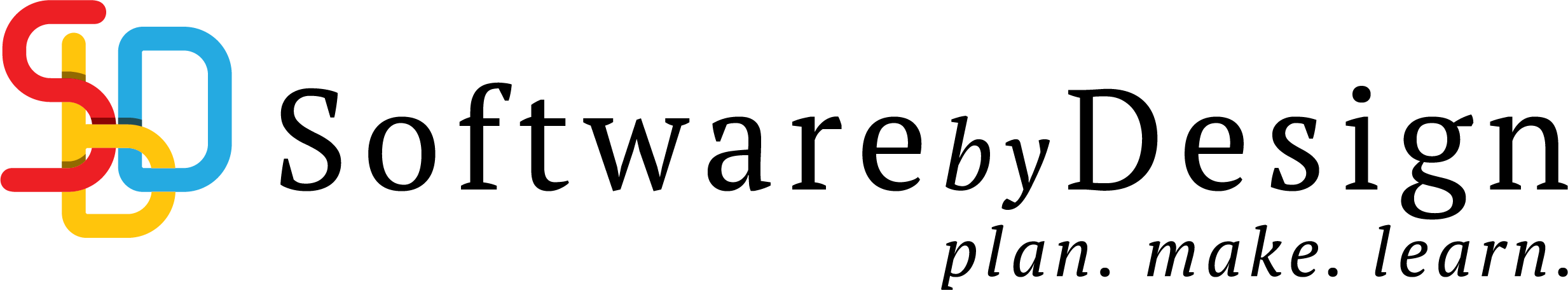# Ross Ch6 Q7 approximate the probability

A 6 sided die, in which each side is equally likely to appear, is repeatedly rolled until the total of all rolls exceeds 400. Approximate the probability that this will require more than 140 rolls.

Let x_i be the number on the face of the die
Let p(x) be the probability of each face = \frac{1}{6}
Let E[X_i] be the expected value of a face

E[X_i] = \sum_1^6 p(x) x = \sum_1^6 \frac{1}{6} x_i = 3.5
Var[x_i] = \sum_1^6 \frac{(x_i-3.5)^2}{6} =\frac{35}{12}

Let E[X] be the sum of the outcomes

E[X] = \sum_1^{140}E[X_i] = 140(3.5) =490

assuming i.i.d ( independent identical distributions )

Var[X] = \sum_1^{140} Var(X_i) = 140\left(\frac{35}{12}\right) = \frac{4900}{12} = \frac{1225}{3}

By Central Limit Theorem, X \sim \mathcal{N}(E[X],Var[X])

(continuity correction)

P(X \leq400) = P(X \leq 400.05)
P\left( \frac{X-490}{\sqrt(\frac{1225}{3})} \leq \frac{400.5-490}{\sqrt(\frac{1225}{3})} \right)

using the general conversion formula

Z=\frac{X-\mu_X}{\sigma_X}
P(Z \leq -4.43)
1 - \phi(4.43) \approx 1-1 = 0